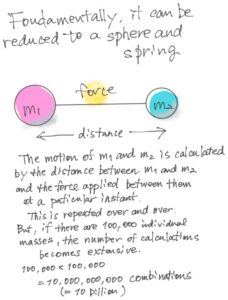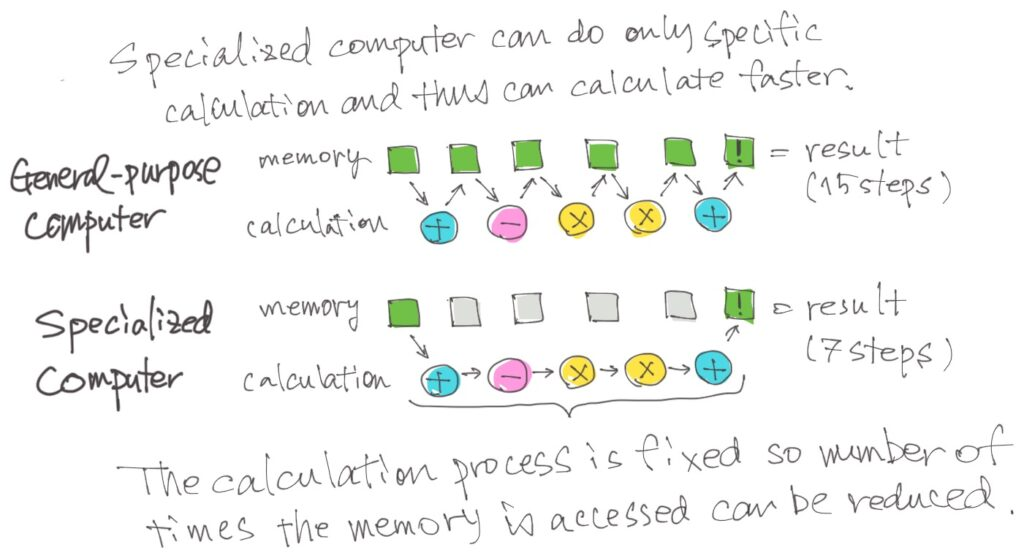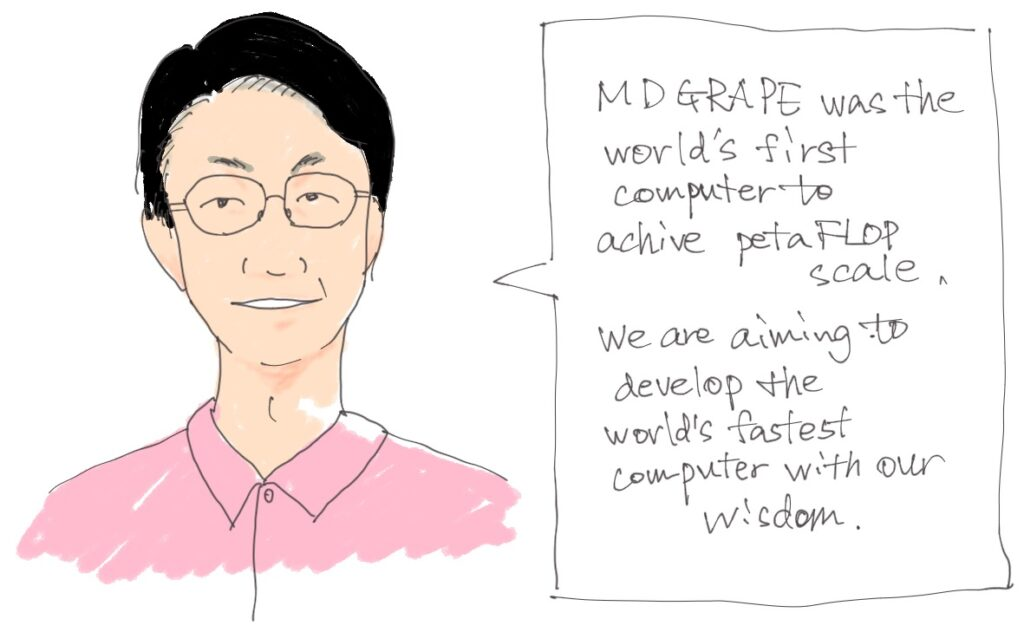This time we have Dr. Yousuke Ohno, who works on the design of MDGRAPE, a computer specialized for running molecular dynamics simulations. If you really think about it, the development of MDGRAPE has been going on since before 1990, which was around the time when Windows 3.1 was finally released. Looking back now, isn’t it amazing to think how its development began in an era with such inefficient computers? With that in mind, let’s hear more from Dr. Ohno, including about that history.

## A computer specialized for simulating molecules

The “MD” in MDGRAPE stands for “molecular dynamics.” That means it’s a computer that simulates molecules, right?

To put it simply, it’s a computer specialized for running simulations that model atoms as spheres and the forces that work between atoms as springs. It examines the shapes that molecules take, and the interactions between them. For instance, there was a simulation of the interactions between novel coronavirus (SARS-CoV-2) proteins and the drugs that bind with them. Watching it may help you understand:

They just keep going round and round.

It’s easiest to understand this when you actually run an MD simulation, but even though atoms stick to one another, the direction of their stickiness varies quite a bit. This video was made to show a framework that is easy to understand, but in the actual simulations, the atoms of every single individual amino acid are calculated.

What’s the approximate time scale?

One computational step is one femtosecond (fsec, 10-15 seconds or one quadrillionth of a second!), and this video shows the activity over one microsecond (μsec, 10-6 seconds or one millionth of a second). For a video of this length, there are one billion calculations performed. Even still, the simulation needs to run longer in order to sufficiently simulate the behavior of proteins.

## Origins in astronomical simulation

The “GRAPE” in MDGRAPE comes from “gravity,” right?

The story starts back in the 1990s, with Dr. Yoshihiro Chikada (honorary professor, National Astronomical Observatory of Japan) at the Nobeyama Radio Observatory in Nagano Prefecture. He came up with the idea that by creating a specialized computer it would be possible to run calculations related to the n-body problem.

Right now, all we can do for the n-body problem is run numerical simulations.

Newton’s law of universal gravitation states that gravity has an infinite range, so if you had, say, one billion stars, there’s gravity working between each pair of them, meaning you have to calculate individually the gravitational force of one billion stars x one billion pairs of stars. The actual calculation programs adopt approximation algorithms to reduce the number of calculated combinations; therefore, even if the number of particles increases by a factor of 10 in commonly used algorithms, the computational complexity does not increase by a factor of 100, rather it is maintained within a factor of approximately 30. There was a proposal that specialized hardware would significantly speed up the calculations for galaxies and groups of stars called globular clusters. Dr. Daiichiro Sugimoto (who was then a professor at the University of Tokyo College of Arts and Sciences) took this proposal, thinking it would be useful in researching the evolution of globular clusters, and set to work on its development. The hardware was named GRAPE, since it subdivides the gravity equation, lines up the many operation circuits, and calculates them in a sort of assembly line called the pipeline method. At first it was only used to calculate gravitational force, but development went on to allow it to be customized for molecular dynamics simulations, which also calculate the forces that act between a pair of particles.

I see. So, on a fundamental level, the gravity problem can also be modeled with a sphere and spring.

MDGRAPE and GRAPE may calculate different phenomena, but both computers are specialized in solving the same problem.## Specialized computers and general-purpose computers

What’s the difference between a computer, being specialized or being general purpose?

Put simply, a specialized computer is specialized for running only certain calculations, with the ability to do other things pruned away. You could say it’s a “thoroughbred.”
Take the calculation of gravitational force, for example. We have the equation for Newton’s law of universal gravitation. You know the one:
\begin{equation}F = G \frac{m_1 m_2}{r^2} \end{equation}
A computer does each of these calculations one by one.

It multiplies the masses $$m_1$$ and $$m_2$$, then squares the distance r…kind of like that?

Yes, like that. In the problem we discussed earlier with one billion stars in a galaxy, it would do that calculation one billion x one billion times. Actually, the distance r is calculated from the coordinates xyz that show the positions of $$m_1$$ and $$m_2$$, so those calculations come in as well.

A general-purpose computer can do those things too, can’t it?

A specialized computer has a circuit just for this calculation physically built onto its chip, and can’t do any other calculations. This allows it to minimize memory use, increasing the speed of calculations.

That sounds pretty niche!

The COVID-19 protein simulation I showed previously has about one million atoms, and that is not the type of problem that could make use of the supercomputer Fugaku’s capability.## The first petaFLOPs computer

Both astronomy and molecules deal with so-called “astronomical numbers.”

It’s the combinations that are the problem. If you increase the number of spheres, the number of combinations increases by the second power.

That seems like it would require an awfully high-spec system.

Yes, but it’s okay for not every single part to be high-spec.

What do you mean by that?

As an example, if the number of particles increases by a factor of ten, the number of calculations for those combinations will increase by a factor of 100. But the computer’s memory only has to retain information on the number of particles increased by ten. So, it is fine if the calculating ability of the computer is increased.
Taking that to the extreme, when MDGRAPE-3 was released, it was the first to achieve petaflop scale. FLOPS (floating point operations per second) are a standard for measuring computer performance, and a petaFLOP means that a computer is able to run one quadrillion (1015) calculations per second. But as a specialized computer, it doesn’t appear on the benchmarks for famous supercomputers, so it isn’t included in TOP500 or other lists like that. Still, at that point, it was theoretically the highest performing computer in the world.

I wish more people knew this fact.

## Accelerating further

In order to run longer simulations, you need to accelerate the calculations even more.

Yes, and when you do that, you run into problems with parts outside the nodes that perform the calculations.
One is data transfer. The transfer of data from the nodes to memory becomes a bottleneck.
As the number of calculations increases, the computer struggles to transfer the data. However, if you shrink the problem enough to let the transfer keep up, you end up just transferring data and doing no calculations. It ends up in a meaningless state for a computer to be in.

I see. You can’t just speed up the calculations and leave it at that.

It’s also not a matter of connecting a bunch of computers together. At this point, it turns into an argument about scaling.

Scaling?

Broadly speaking, there is an issue of strong versus weak scaling.For example, if 100 cooks use 100 frying pans, they can make 100 omelets in the same time it takes them to make one. This is called “weak scaling.” But this doesn’t mean that they can make an omelet any faster. By contrast, strong scaling allows you to make one omelet in one-one hundredth of the time. It requires a different approach.

Still, even with 100 frying pans, you can’t make an omelet 100 times the standard size. After you’ve made a frying pan that’s 100 times bigger, the question of whether it works is the difference between supercomputers like Fugaku and simply connecting PCs together.

What’s coming up next?

Right now, we’re working on splitting calculations in a somewhat modular way, and designing the next computer as we improve the algorithms.

I really learned a lot! I hope that MDGRAPE will become the world’s fastest computer again!### Postscript

After hearing Dr. Ohno’s riveting explanation, I thought it was a real shame that specialized computers like MDGRAPE are relatively obscure. Manufacture of the specialized chips used in these computers is also necessary, and he also told me the struggles of that process (namely their small production lots), but the conversation got so long that we couldn’t fit it into this article. How sad…